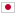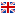Contest Duration: - (local time) (300 minutes) Back to Home
D - Janken Master/Time Limit: 2 sec / Memory Limit: 512 MB

### Problem Statement

You are supposed to play the rock-paper-scissors game. There are $N$ players including you.

This game consists of multiple rounds. While the rounds go, the number of remaining players decreases. In each round, each remaining player will select an arbitrary shape independently. People who show rocks win if all of the other people show scissors. In this same manner, papers win rocks, scissors win papers. There is no draw situation due to the special rule of this game: if a round is tied based on the normal rock-paper-scissors game rule, the player who has the highest programming contest rating (this is nothing to do with the round!) will be the only winner of the round. Thus, some players win and the other players lose on each round. The losers drop out of the game and the winners proceed to a new round. They repeat it until only one player becomes the winner.

Each player is numbered from $1$ to $N$. Your number is $1$. You know which shape the other $N-1$ players tend to show, that is to say, you know the probabilities each player shows rock, paper and scissors. The $i$-th player shows rock with $r_i$% probability, paper with $p_i$% probability, and scissors with $s_i$% probability. The rating of programming contest of the player numbered $i$ is $a_i$. There are no two players whose ratings are the same. Your task is to calculate your probability to win the game when you take an optimal strategy based on each player's tendency and rating.

### Input

The input consists of a single test case formatted as follows.

$N$ $a_1$ $a_2 \ r_2 \ p_2 \ s_2$ $\vdots$ $a_N \ r_N \ p_N \ s_N$

The first line consists of a single integer $N \ (2 \le N \le 14)$. The second line consists of a single integer $a_1 \ (1 \le a_1 \le N)$. The $(i+1)$-th line consists of four integers $a_i$, $r_i$, $p_i$ and $s_i$ ($1 \le a_i \le N$, $0 \le r_i, p_i, s_i \le 100$, $r_i + p_i + s_i = 100$) for $i = 2, \ldots, N$. It is guaranteed that $a_1, \ldots, a_N$ are pairwise distinct.

### Output

Print the probability to win the game in one line. Your answer will be accepted if its absolute or relative error does not exceed $10^{-6}$.

### Sample Input 1

2
2
1 40 40 20


### Output for Sample Input 1

0.8


Since you have the higher rating than the other player, you will win the game if you win or draw in the first round.

### Sample Input 2

2
1
2 50 50 0


### Output for Sample Input 2

0.5


You must win in the first round.

### Sample Input 3

3
2
1 50 0 50
3 0 0 100


### Output for Sample Input 3

1


In the first round, your best strategy is to show a rock. You will win the game with 50% in this round. With the other 50%, you and the second player proceed to the second round and you must show a rock to win the game.

### Sample Input 4

3
2
3 40 40 20
1 30 10 60


### Output for Sample Input 4

0.27


### Sample Input 5

4
4
1 34 33 33
2 33 34 33
3 33 33 34


### Output for Sample Input 5

0.6591870816